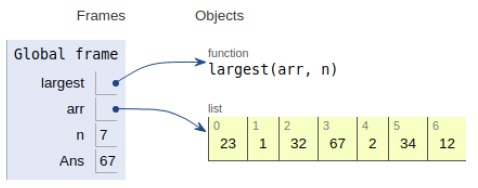# Python Program to find the largest element in an array

Problem statement − We are given an array, we need to calculate the largest element of the array.

Here we use the bruteforce approach in which we compute the largest element by traversing the whole loop and get the element.

We can observe the implementation below.

## Example

Live Demo

# largest function
def largest(arr,n):
#maximum element
max = arr
# traverse the whole loop
for i in range(1, n):
if arr[i] > max:
max = arr[i]
return max
# Driver Code
arr = [23,1,32,67,2,34,12]
n = len(arr)
Ans = largest(arr,n)
print ("Largest element given in array is",Ans)

## Output

Largest in given array is 67All the variables are declared in global form as shown above

## Conclusion

In this article, we have learned how to get the largest element from the array.